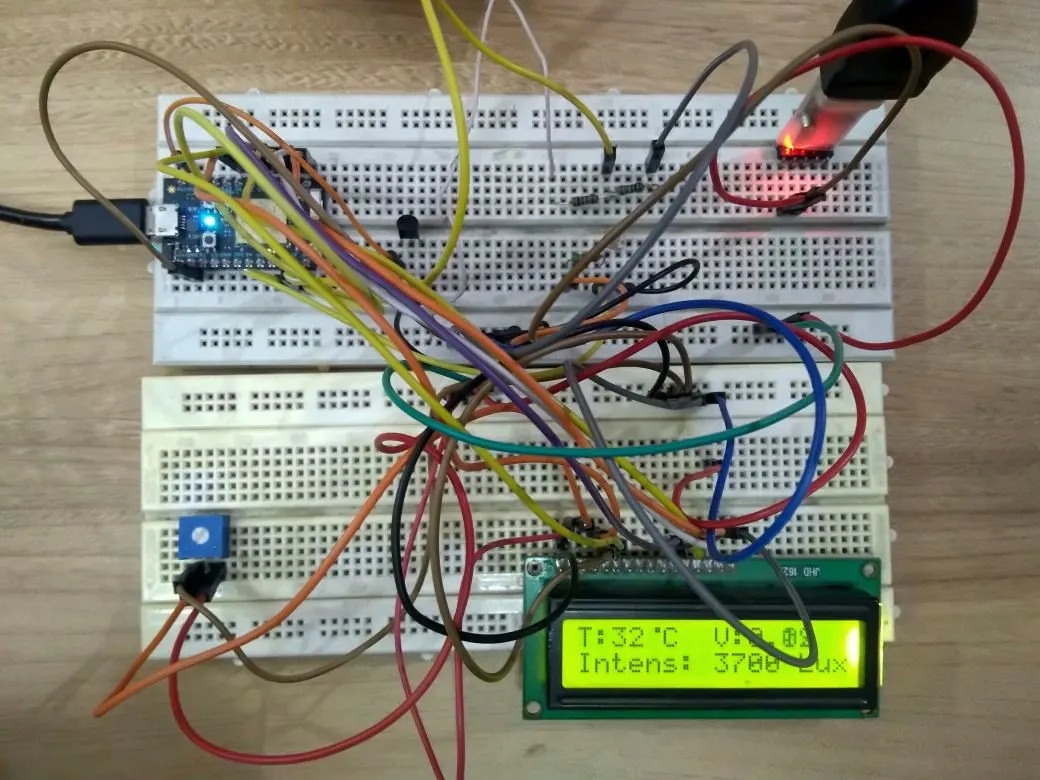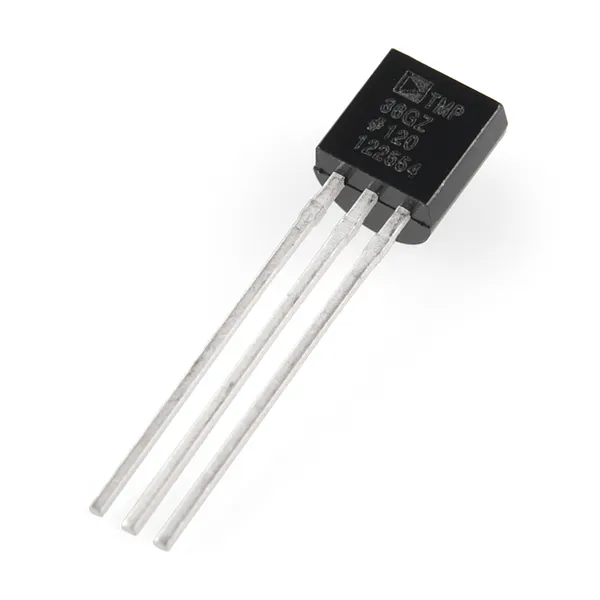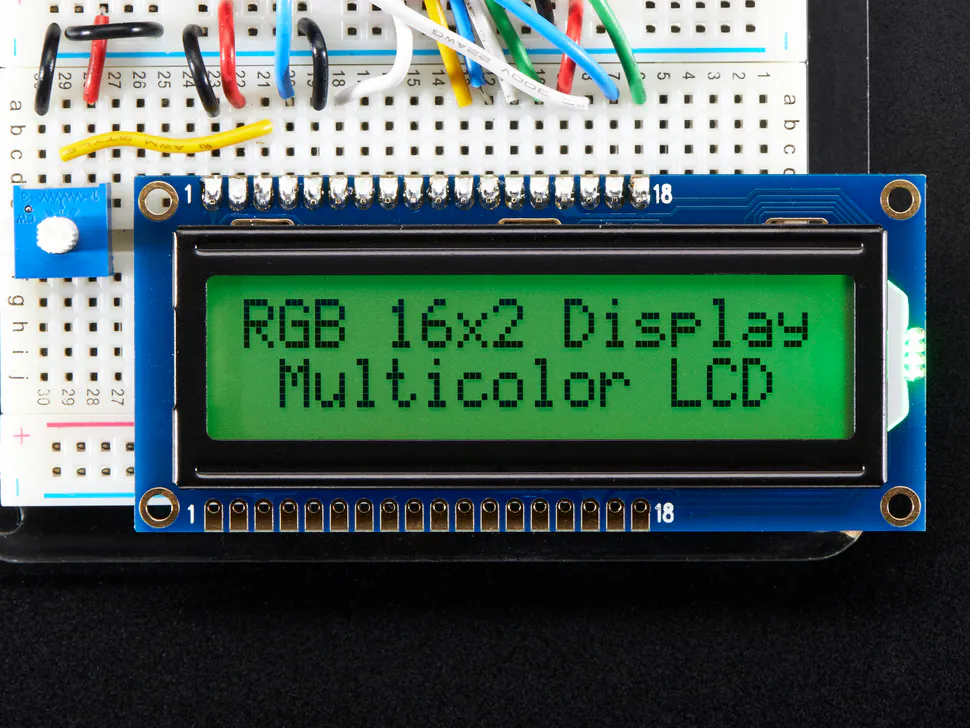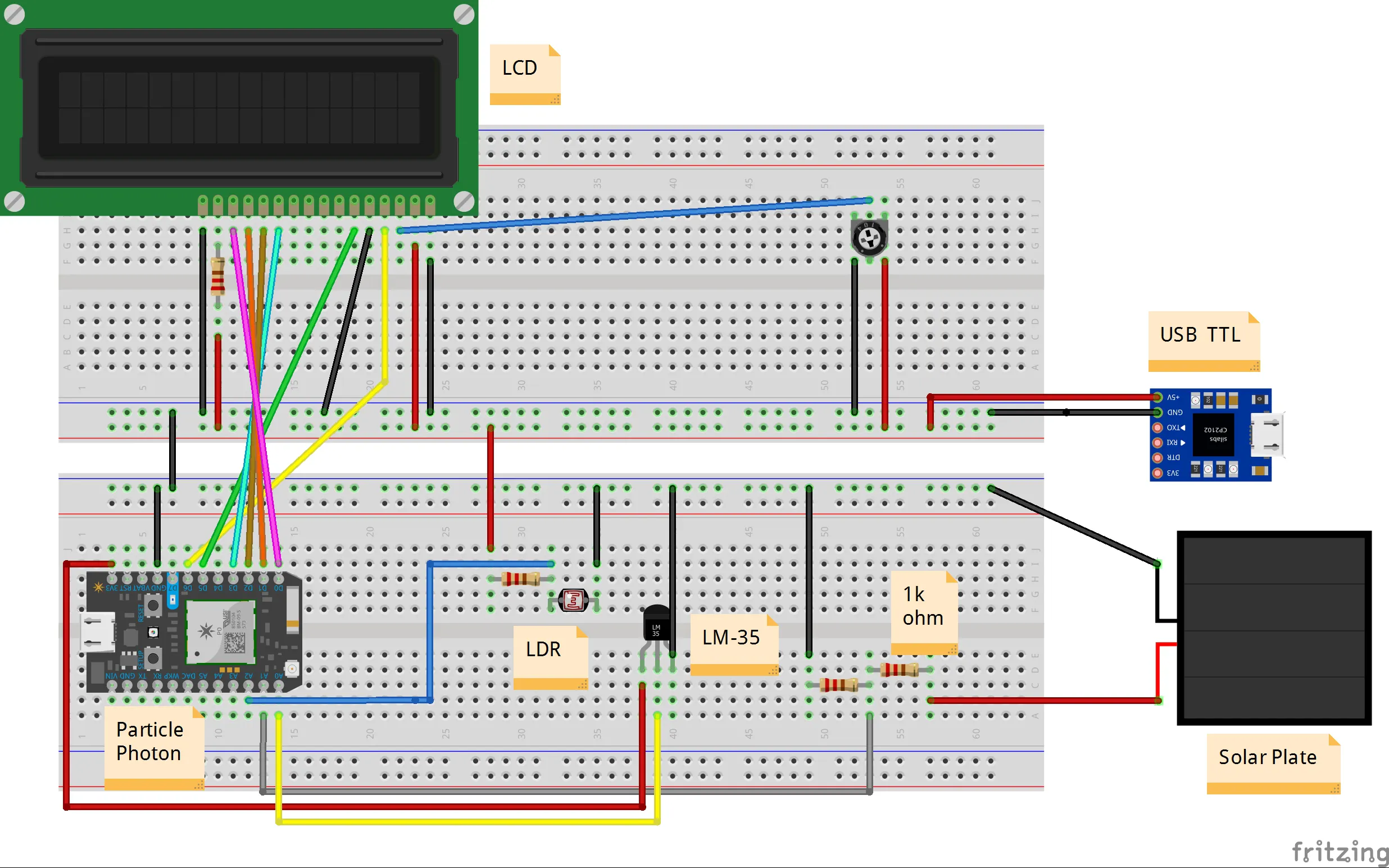# Solar Panel Monitoring

The aim is to supervise solar photovoltaic power generation to enhance the performance, monitoring and maintenance of the solar plant.

BeginnerFull instructions provided1 hour3,491## Things used in this project

### Hardware componentsParticle Photon
×1
 Solar plate
×1
 Light Dependent Resistor (LDR)
×1Temperature Sensor
×1Adafruit RGB Backlight LCD - 16x2
×1Resistor 1k ohm
×3

## Schematics

### Circuit Diagram of solar panel monitoring## Code

### Solar panel monitoring

Objective-C
To be used in particle Web IDE
```#include <LiquidCrystal.h> // library for LCD

LiquidCrystal lcd(6, 5, 3, 2, 1, 0); // LCD connection

/*
LCD ==> Particle
RS ==> D6
Enable ==> D5
DB4 ==> D3
DB5 ==> D2
DB6 ==> D1
DB7 ==> D0

*/

float Temperature = 0;

float temp1 = 0;

float volts = 0;

float value = 0;

float volt  = 0;

float lux  = 0;

byte degree =

//array of byte is declared and initialized to display the special character //degree symbol on the LCD.

{

0b00011,

0b00011,

0b00000,

0b00000,

0b00000,

0b00000,

0b00000,

0b00000

};

void setup()

{

Serial.begin(9600); // baudrate 9600

lcd.begin(16,2); //  Initialize the LCD

lcd.createChar(1, degree);

lcd.setCursor(0,0);

lcd.print(" Solar Panel ");

lcd.setCursor(0,1);

lcd.print("  Monitoring   ");

delay(2000);

lcd.clear();

lcd.setCursor(0,0);

lcd.print("        For ");

lcd.setCursor(0,1);

lcd.print("ParticleContest");

delay(2000);

lcd.clear();

pinMode(A0, INPUT);  // interface LM-35 to A0 pin of particle

pinMode(A1, INPUT);  // interface solar plate to A1 pin of particle

pinMode(A2, INPUT);  // interface LDR to A2 pin of particle

}

void loop()

{

/*---------Temperature-------*/

// 3.3V/4095= 0.80

Temperature = (Temperature/10);

// LM35 gives output of 10mv/°C

delay(10);

/*---------Voltage----------*/

volts= (temp1*3.3)/4095;

//volts= (temp1/511.5)*3;

delay(10);

/*-----Light Intensity------*/

volt= (lux/4095*100);

//volt=(value/1023.0)*5;

//lux=((2500/volt)-500)/3.3;

delay(10);

/*------Display Result------*/

lcd.clear();

lcd.setCursor(0,0);

lcd.print("T:");

lcd.print((int)Temperature);

lcd.write(1);

lcd.print("C");

lcd.setCursor(8,0);

lcd.print("V:");

lcd.print(volts);

lcd.setCursor(0,1);

lcd.print("Intens: ");

lcd.print((int)lux);

lcd.print(" Lux");

//Serial.println((int)Temp);

// Serial.println(volts);

// Serial.println((int)lux);

//delay(500);

// To see events on console

Particle.publish("Voltage", String(volts));

Particle.publish("Temperature", String(Temperature));

Particle.publish("Light Intensity", String(lux));

delay(2000);

}
```

## Credits

### Ruchir Sharma

12 projects • 171 followers